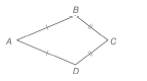Chapter 4.2, Problem 33EElementary Geometry For College St...

7th Edition
Alexander + 2 others
ISBN: 9781337614085

Solutions

Chapter
SectionElementary Geometry For College St...

7th Edition
Alexander + 2 others
ISBN: 9781337614085
Textbook Problem

For Exercises 32 to 35, consider kite A B C D with A B ¯ ≅ A D ¯ and B C ¯ ≅ D C ¯ .For kite A B C D , m ∠ C = m ∠ - 30 while m ∠ A = m ∠ B - 50 . Find m ∠ B .

To determine

To find:

mB

Explanation

Calculation:

Given,

The kite ABCD with

mC=mB-30, and

mA=mB-50.

In any quadrilateral, the sum of all the four interior angles is 360°

Thus,

A+B+C+D=360°

But, for the kite ABCD

B=D

A+B+C+B=360°

A+2B+C=360°

Now,

B-50°+2B+B-30°=360°

Still sussing out bartleby?

Check out a sample textbook solution.

See a sample solution

The Solution to Your Study Problems

Bartleby provides explanations to thousands of textbook problems written by our experts, many with advanced degrees!

Get Started

11. Find y’ if .

Mathematical Applications for the Management, Life, and Social Sciences

Proof Prove that if limxcf(x)=0, then limxc|f(x)|=0.

Calculus: Early Transcendental Functions (MindTap Course List)

Sometimes, Always, or Never: The antiderivative of an elementary function is elementary.

Study Guide for Stewart's Single Variable Calculus: Early Transcendentals, 8th

Explain how you would find the inverse of a nonsingular matrix.

Finite Mathematics for the Managerial, Life, and Social Sciences